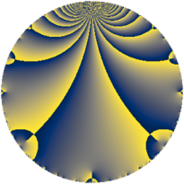# Properties

 Label 7448.2.a.wLevel $7448$ Weight $2$ Character orbit 7448.a Self dual yes Analytic conductor $59.473$ Analytic rank $1$ Dimension $2$ CM no Inner twists $1$

# Related objects

## Newspace parameters

 Level: $$N$$ $$=$$ $$7448 = 2^{3} \cdot 7^{2} \cdot 19$$ Weight: $$k$$ $$=$$ $$2$$ Character orbit: $$[\chi]$$ $$=$$ 7448.a (trivial)

## Newform invariants

 Self dual: yes Analytic conductor: $$59.4725794254$$ Analytic rank: $$1$$ Dimension: $$2$$ Coefficient field: $$\Q(\sqrt{57})$$ Defining polynomial: $$x^{2} - x - 14$$ Coefficient ring: $$\Z[a_1, \ldots, a_{11}]$$ Coefficient ring index: $$1$$ Twist minimal: no (minimal twist has level 1064) Fricke sign: $$1$$ Sato-Tate group: $\mathrm{SU}(2)$

## $q$-expansion

Coefficients of the $$q$$-expansion are expressed in terms of $$\beta = \frac{1}{2}(1 + \sqrt{57})$$. We also show the integral $$q$$-expansion of the trace form.

 $$f(q)$$ $$=$$ $$q -2 q^{3} - q^{5} + q^{9} +O(q^{10})$$ $$q -2 q^{3} - q^{5} + q^{9} + ( -1 - \beta ) q^{11} + 2 q^{13} + 2 q^{15} + ( 4 - \beta ) q^{17} - q^{19} + ( -1 + 2 \beta ) q^{23} -4 q^{25} + 4 q^{27} -4 q^{29} -4 q^{31} + ( 2 + 2 \beta ) q^{33} + 2 q^{37} -4 q^{39} + ( 2 + 2 \beta ) q^{41} - q^{43} - q^{45} + ( 1 + \beta ) q^{47} + ( -8 + 2 \beta ) q^{51} + 2 \beta q^{53} + ( 1 + \beta ) q^{55} + 2 q^{57} -4 q^{59} + ( 9 - \beta ) q^{61} -2 q^{65} + ( 2 - 4 \beta ) q^{69} + ( 4 - 2 \beta ) q^{71} + ( 7 + \beta ) q^{73} + 8 q^{75} + ( -6 - 2 \beta ) q^{79} -11 q^{81} + ( 5 - 2 \beta ) q^{83} + ( -4 + \beta ) q^{85} + 8 q^{87} + ( 10 - 2 \beta ) q^{89} + 8 q^{93} + q^{95} + ( 2 - 2 \beta ) q^{97} + ( -1 - \beta ) q^{99} +O(q^{100})$$ $$\operatorname{Tr}(f)(q)$$ $$=$$ $$2 q - 4 q^{3} - 2 q^{5} + 2 q^{9} + O(q^{10})$$ $$2 q - 4 q^{3} - 2 q^{5} + 2 q^{9} - 3 q^{11} + 4 q^{13} + 4 q^{15} + 7 q^{17} - 2 q^{19} - 8 q^{25} + 8 q^{27} - 8 q^{29} - 8 q^{31} + 6 q^{33} + 4 q^{37} - 8 q^{39} + 6 q^{41} - 2 q^{43} - 2 q^{45} + 3 q^{47} - 14 q^{51} + 2 q^{53} + 3 q^{55} + 4 q^{57} - 8 q^{59} + 17 q^{61} - 4 q^{65} + 6 q^{71} + 15 q^{73} + 16 q^{75} - 14 q^{79} - 22 q^{81} + 8 q^{83} - 7 q^{85} + 16 q^{87} + 18 q^{89} + 16 q^{93} + 2 q^{95} + 2 q^{97} - 3 q^{99} + O(q^{100})$$

## Embeddings

For each embedding $$\iota_m$$ of the coefficient field, the values $$\iota_m(a_n)$$ are shown below.

For more information on an embedded modular form you can click on its label.

Label $$\iota_m(\nu)$$ $$a_{2}$$ $$a_{3}$$ $$a_{4}$$ $$a_{5}$$ $$a_{6}$$ $$a_{7}$$ $$a_{8}$$ $$a_{9}$$ $$a_{10}$$
1.1
 4.27492 −3.27492
0 −2.00000 0 −1.00000 0 0 0 1.00000 0
1.2 0 −2.00000 0 −1.00000 0 0 0 1.00000 0
 $$n$$: e.g. 2-40 or 990-1000 Significant digits: Format: Complex embeddings Normalized embeddings Satake parameters Satake angles

## Atkin-Lehner signs

$$p$$ Sign
$$2$$ $$1$$
$$7$$ $$1$$
$$19$$ $$1$$

## Inner twists

This newform does not admit any (nontrivial) inner twists.

## Twists

By twisting character orbit
Char Parity Ord Mult Type Twist Min Dim
1.a even 1 1 trivial 7448.2.a.w 2
7.b odd 2 1 7448.2.a.be 2
7.c even 3 2 1064.2.q.l 4

By twisted newform orbit
Twist Min Dim Char Parity Ord Mult Type
1064.2.q.l 4 7.c even 3 2
7448.2.a.w 2 1.a even 1 1 trivial
7448.2.a.be 2 7.b odd 2 1

## Hecke kernels

This newform subspace can be constructed as the intersection of the kernels of the following linear operators acting on $$S_{2}^{\mathrm{new}}(\Gamma_0(7448))$$:

 $$T_{3} + 2$$ $$T_{5} + 1$$ $$T_{11}^{2} + 3 T_{11} - 12$$ $$T_{13} - 2$$ $$T_{17}^{2} - 7 T_{17} - 2$$

## Hecke characteristic polynomials

$p$ $F_p(T)$
$2$ $$T^{2}$$
$3$ $$( 2 + T )^{2}$$
$5$ $$( 1 + T )^{2}$$
$7$ $$T^{2}$$
$11$ $$-12 + 3 T + T^{2}$$
$13$ $$( -2 + T )^{2}$$
$17$ $$-2 - 7 T + T^{2}$$
$19$ $$( 1 + T )^{2}$$
$23$ $$-57 + T^{2}$$
$29$ $$( 4 + T )^{2}$$
$31$ $$( 4 + T )^{2}$$
$37$ $$( -2 + T )^{2}$$
$41$ $$-48 - 6 T + T^{2}$$
$43$ $$( 1 + T )^{2}$$
$47$ $$-12 - 3 T + T^{2}$$
$53$ $$-56 - 2 T + T^{2}$$
$59$ $$( 4 + T )^{2}$$
$61$ $$58 - 17 T + T^{2}$$
$67$ $$T^{2}$$
$71$ $$-48 - 6 T + T^{2}$$
$73$ $$42 - 15 T + T^{2}$$
$79$ $$-8 + 14 T + T^{2}$$
$83$ $$-41 - 8 T + T^{2}$$
$89$ $$24 - 18 T + T^{2}$$
$97$ $$-56 - 2 T + T^{2}$$